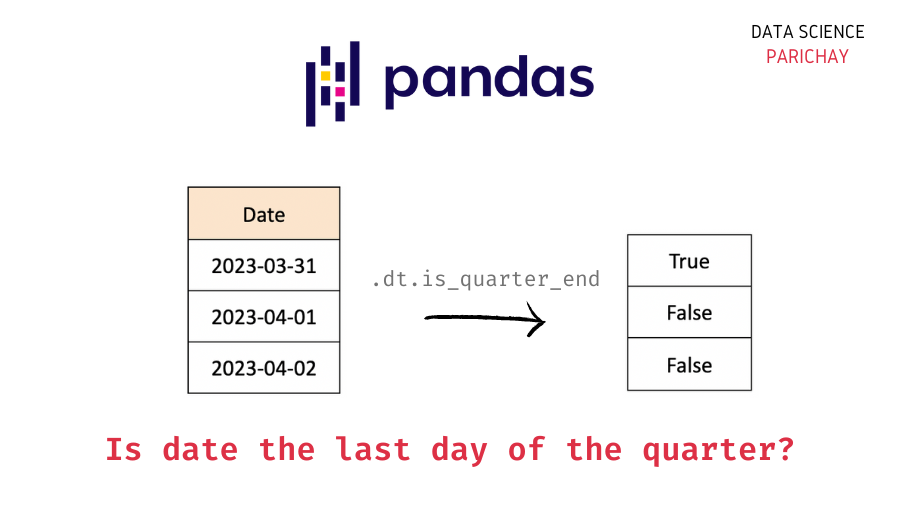# Pandas – Check If Date is the Last Day of a Quarter

The datetime type in pandas comes with a number of useful built-in functions to get additional information about the given date. In this tutorial, we will look at how to check if a date in a pandas dataframe is the last day of a quarter or not with the help of some examples.

## Using the `is_quarter_end` attribute

You can use `is_quarter_end` attribute on a pandas series (or a column in a dataframe) containing datetime values with the help the `.dt` accessor to check if each date is the last date of a quarter or not.

The following is the syntax –

`df["Date"].dt.is_quarter_end`

It returns a boolean pandas series where `True` represents that the corresponding date is the end date of the quarter and `False` represents that it’s not.

## Examples

Let’s now look at some examples of using the above syntax.

First, we will create a sample dataframe to apply the above method.

```import pandas as pd

# create a dataframe
df = pd.DataFrame({
"Date": pd.date_range("2023-03-26", "2023-04-04"),
"Sales": [10, 12, 7, 17, 21, 5, 11, 13, 14, 8]
})

# display the dataframe
df```

Output:

Here, we created a dataframe with the date level sales data for an ecommerce website. Notice, we used the pandas `date_range()` function to get datetime values from “2022-03-26” to “2022-04-04” (both inclusive).

📚 Data Science Programs By Skill Level

Introductory

Intermediate ⭐⭐⭐

🔎 Find Data Science Programs 👨‍💻 111,889 already enrolled

Disclaimer: Data Science Parichay is reader supported. When you purchase a course through a link on this site, we may earn a small commission at no additional cost to you. Earned commissions help support this website and its team of writers.

Let’s now check whether the dates in the “Date” represent the last day of a quarter or not.

`df["Date"].dt.is_quarter_end`

Output:

```0    False
1    False
2    False
3    False
4    False
5     True
6    False
7    False
8    False
9    False
Name: Date, dtype: bool```

We get a pandas series of boolean values. Notice that we get `True` for the date “2023-03-31” which, in fact, is the last day of a quarter (January to March).

Let’s add the result from the `is_quarter_end` attribute as an additional column in the above dataframe.

```# add new column
df["Is Last Day of Quarter"] = df["Date"].dt.is_quarter_end
# display the dataframe
df```

Output:

You might also be interested in –

•